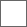• 29回复贴，共1

### 求教问题#include <stdio.h>
int main(void)
{
FILE *fp1,*fp2;
signed char c;
fp1=fopen("a.txt","r"); /*打开源文件*/
fp2=fopen("b.txt","w"); /*打开将写入的文件*/
while ((c=fgetc(fp1))!=EOF) /*将源文件fp1的内容转存(复制)到目标文件fp2中*/
fputc(c,fp2);
fclose(fp1); /*关闭文件*/
fclose(fp2);
return 0;
}//这是有效的，你参考吧
#include <stdio.h>
int main() {
signed char c;
FILE *f1 = fopen("a.txt", "rb");
FILE *f2 = fopen("b.txt", "wb");
while ((c = fgetc(f1)) != EOF)
fputc(c, f2);
fclose(f1);
fclose(f2);
return 0;
}//你把这个运行一下截个图
#include <stdio.h>
#include <stdlib.h>
int main(void) {
system("cat a.txt");
FILE *fp1, *fp2;
signed char c;
fp1 = fopen("a.txt", "r"); /*打开源文件*/
fp2 = fopen("b.txt", "w"); /*打开将写入的文件*/
while ((c = fgetc(fp1)) != EOF) /*将源文件fp1的内容转存(复制)到目标文件fp2中*/
fputc(c, fp2);
fclose(fp1); /*关闭文件*/
fclose(fp2);
system("cat b.txt");
return 0;
}#### 扫二维码下载贴吧客户端